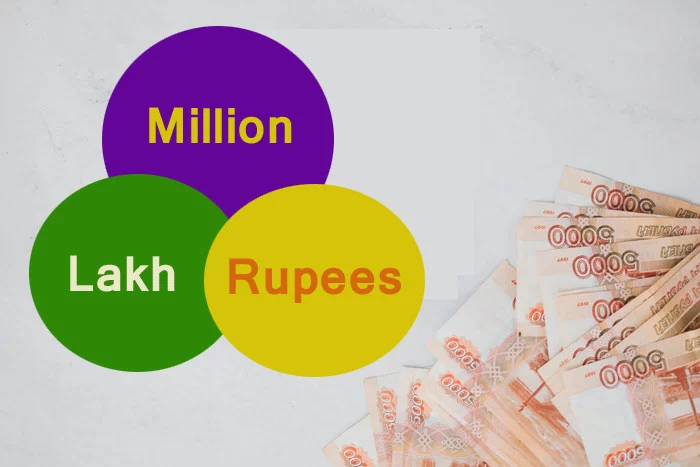# Millions to Lakhs and RupeesA million isn't just a number, it’s a thousand thousands. This is what the word “million” means. It may be hard to believe but there are people even today who don’t know how many zeros there are in a million.

One million is a natural number following 999,999 and preceding 1,000,001. The word "million" is written as "1,000,000" in America and most English-speaking countries use this numeric system.

### How Many Lakhs is 1 Million

When talking about a large amount, we often use the words lakhs and millions.

The Indian numbering system has different place values of digits compared to the International numbering system. It goes like - Ones, Tens, Hundreds, Thousands, Ten Thousand, Lakhs, Ten Lakhs, Crores, and so on.

In the International numbering system, the place value of digits are as follows - Ones, Tens, Hundreds, Thousands, Ten Thousand, Hundred Thousand, Millions, Billions, and so on.

When you convert the amount from millions to rupees, this is what you get:

### How Many Rupees is 1 Million

In the International numeral system, large numbers are represented using "million". Let us see the value of millions in rupees given in the below table:

Millions

Rupees

1

1000000

2

2000000

10

10000000

50

50000000

60

60000000

70

70000000

100

100000000

1000

1000000000

### How much is 1 Million?

In India, the amount of money is denoted by ten, hundred, thousand, etc. If it is a large sum of money, the amount is described in millions.

Numerically, it is represented as:

1 Million = 1000000 rupees

1 Million = 10 lakhs

1 Million = 0.1 crore

### How many crores is 1 Million?

The currency from millions to crores is done using a conversion method. With this method, you can convert numbers from the International number system to the Indian number system. The place values of the digits are extremely different in the two different numbering systems.

Thus, the expression of numbers from million to crores is given as:

1 Million = 0.1 crore

10 Millions = 1 crore

20 Millions = 2 crores

100 Millions = 10 crores

### FAQs on One Million

#### How much is the value of 1 million?

1 million is valued as 10 lakhs in Indian rupees. It is abbreviated as m. Example - 2 million can be represented as 2m

#### What do you mean by 1 billion?

One billion is equal to 100 crores in Indian rupees. In terms of millions, the value is 1000 million.